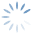Help OnLine | Options Portfolio – Cash Flow / Risk
3395

# Options Portfolio – Cash Flow / Risk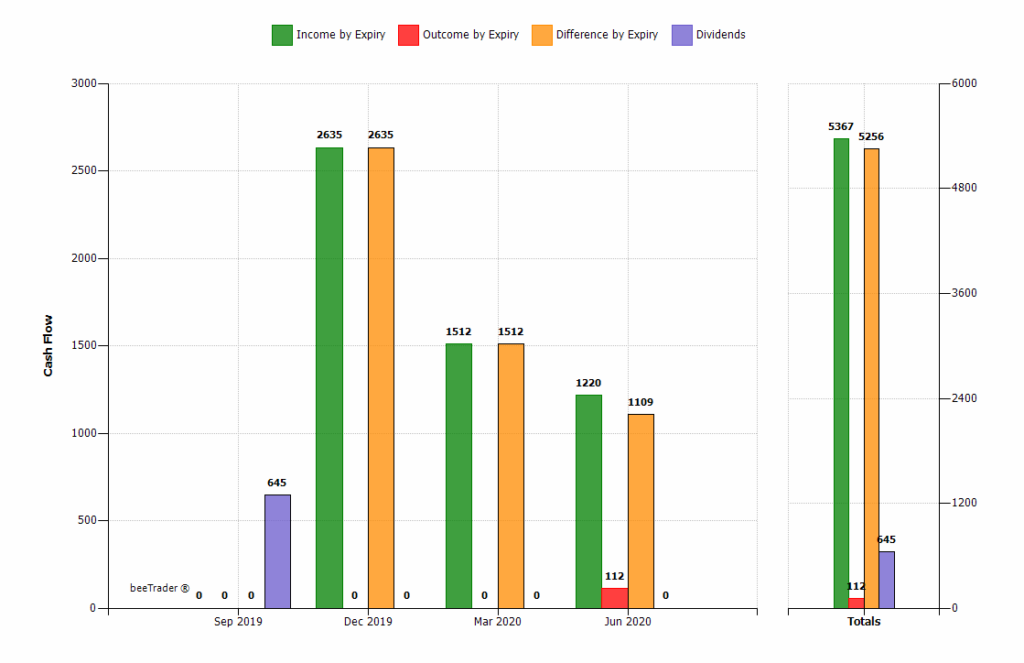Cash Flow:

histogram chart showing the sum of the collected premiums, the prizes spent and the relative difference of the strategies in the portfolio. On the left the prizes for each deadline (in the case in the example are December 2018), on the right the total sum.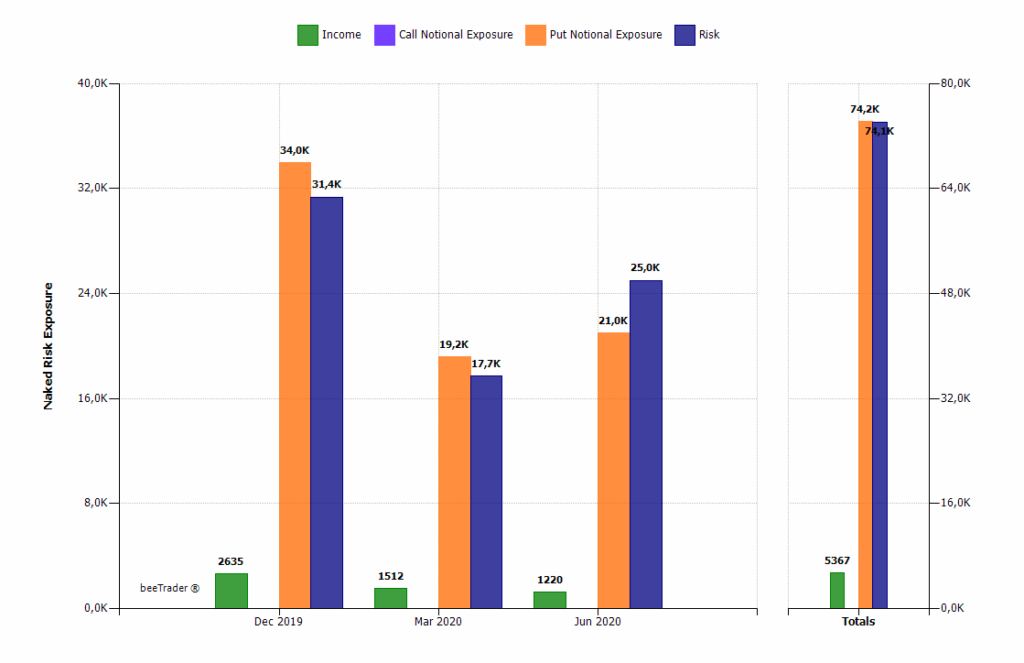Exposure & Risk (by Expiry):

histogram chart that displays, for each expiry, the collected premiums (Income), the notional exposure of the Call options, the notional exposure of the Put options, and the risk. This risk is calculated as the maximum risk of the strategy if it is not infinite, or maximum risk calculated with the montecarlo simulation (in this case the histogram becomes blurred).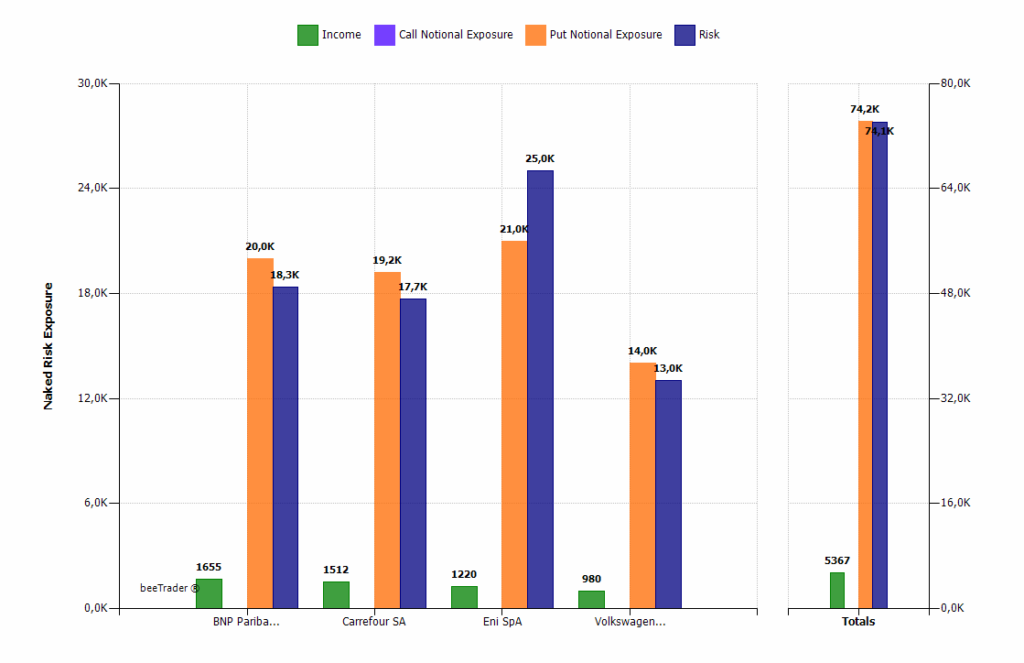Exposure & Risk (by Underlying):

histogram chart that displays, for each underlying, the premiums received (Income), the notional exposure of the Call options, the notional exposure of the Put options, and the risk. This risk is calculated as the maximum risk of the strategy if it is not infinite, or maximum risk calculated with the montecarlo simulation (in this case the histogram becomes blurred).Commitment Sectors:

pie chart that represents the commitment of the portfolio divided by sector of belonging of the underlying.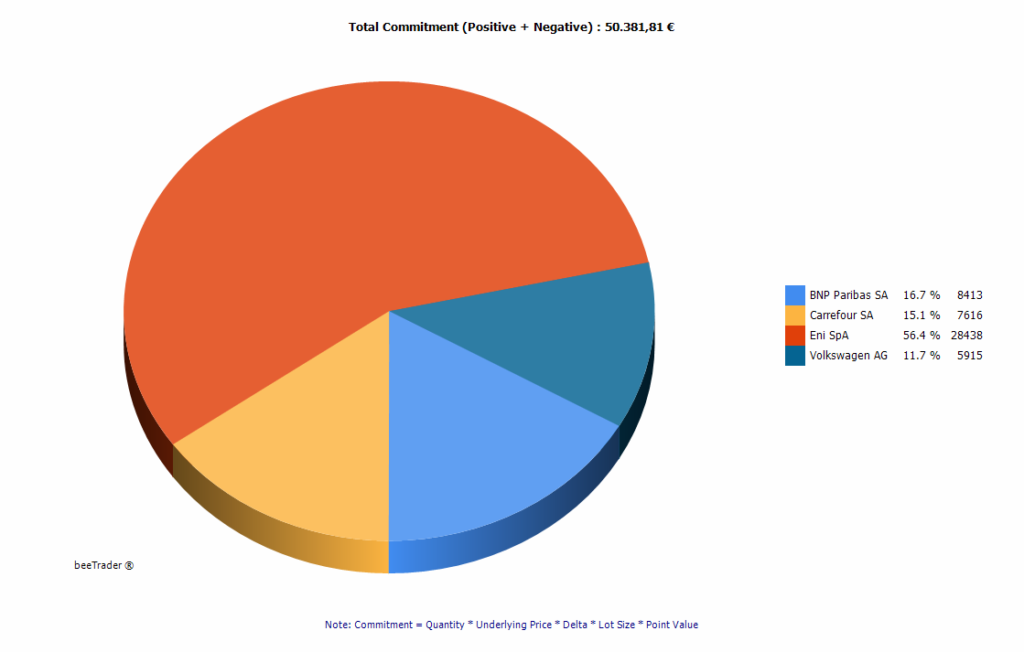Commitment Underlying:

pie chart that represents the commitment of the portfolio divided by underlying.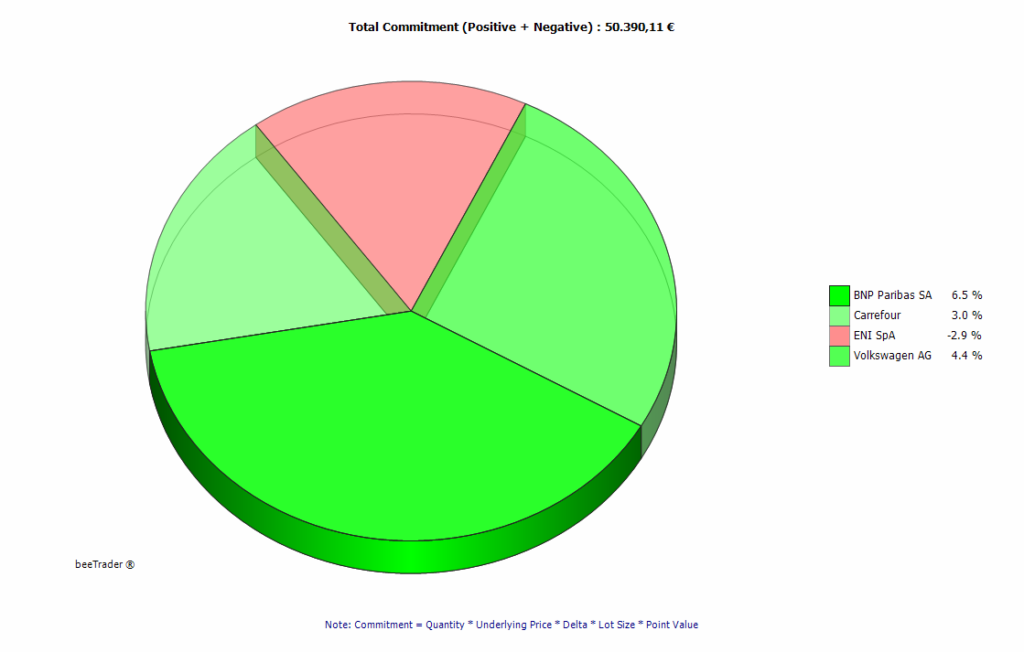Commitment Performance:

pie chart that represents the commitment of the portfolio divided by strategy. The coloring of the sectors is attributed on the basis of the At Now% value, which is the relationship between At Now and Max Profit of the strategy. Green therefore means that the value at now is in the prices of the max profit, red that the value at now is very far from the max profit. Between the two extreme values the other strategies are colored in order to have an immediate perception of which strategy is going well and which are not.